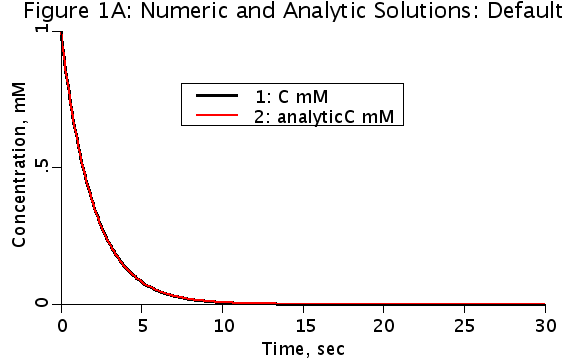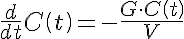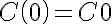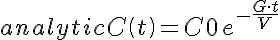Comp1DecayPlus

Model number
0281

One compartment model with decay of substance

DescriptionThis is a one compartment model with a single substrate, C. C decays with rate constant G. Additionally, this model includes a tutorial on sensitivity analysis and optimization. Compartmental models are based on mass balance equations. The compartment has a volume, V, and a time-varying concentration of a substance, C(t). An underlying assumption of compartmental models is that the material in the compartment is instantaneously well mixed. The total amount of material, Q(t), is given by the equation Q(t)=V*C(t). The time rate of change of the amount of material is given by the derivative, dQ(t)/dt. If the volume is constant through time, then dQ(t)/dt=V*dC(t)/dt. The rate of change of the material in the volume equals the SUM OF THE SOURCES MINUS THE SINKS for the material. This is a model for exponential decay (and also growth). It provides a numeric solution and an analytic solution. The independent variable is named ?t? standing for time. The sink for the material is the exponential decay. It removes material from the system.

Further reading:     Understanding the Comp1DecayPlus Model

Equations

Ordinary Differential EquationInitial ConditionAnalytic SolutionThe equations for this model may be viewed by running the JSim model applet and clicking on the Source tab at the bottom left of JSim's Run Time graphical user interface. The equations are written in JSim's Mathematical Modeling Language (MML). See the Introduction to MML and the MML Reference Manual. Additional documentation for MML can be found by using the search option at the Physiome home page.

References

None.

Key terms
Transport physiology
compartment
compartmental
decay
one
single
sensitivity
optimization
tutorial
Acknowledgements

Please cite https://www.imagwiki.nibib.nih.gov/physiome in any publication for which this software is used and send one reprint to the address given below:
The National Simulation Resource, Director J. B. Bassingthwaighte, Department of Bioengineering, University of Washington, Seattle WA 98195-5061.

Model development and archiving support at https://www.imagwiki.nibib.nih.gov/physiome provided by the following grants: NIH U01HL122199 Analyzing the Cardiac Power Grid, 09/15/2015 - 05/31/2020, NIH/NIBIB BE08407 Software Integration, JSim and SBW 6/1/09-5/31/13; NIH/NHLBI T15 HL88516-01 Modeling for Heart, Lung and Blood: From Cell to Organ, 4/1/07-3/31/11; NSF BES-0506477 Adaptive Multi-Scale Model Simulation, 8/15/05-7/31/08; NIH/NHLBI R01 HL073598 Core 3: 3D Imaging and Computer Modeling of the Respiratory Tract, 9/1/04-8/31/09; as well as prior support from NIH/NCRR P41 RR01243 Simulation Resource in Circulatory Mass Transport and Exchange, 12/1/1980-11/30/01 and NIH/NIBIB R01 EB001973 JSim: A Simulation Analysis Platform, 3/1/02-2/28/07.